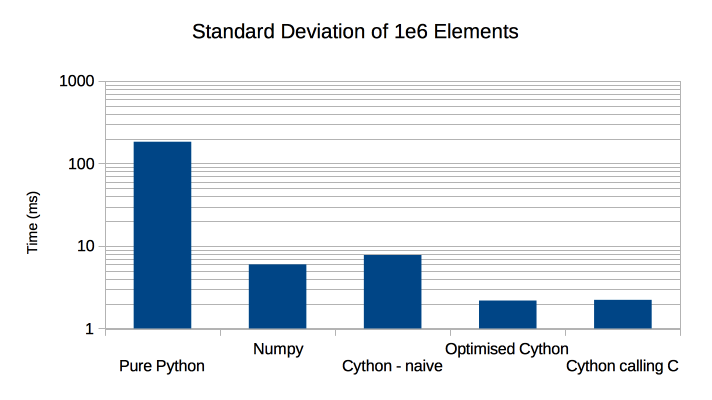# The Performance of Python, Cython and C on a Vector¶

Lets look at a real world numerical problem, namely computing the standard deviation of a million floats using:

• Pure Python (using a list of values).
• Numpy.
• Cython expecting a numpy array - naive
• Cython expecting a numpy array - optimised
• C (called from Cython)

The pure Python code looks like this, where the argument is a list of values:

```# File: StdDev.py

import math

def pyStdDev(a):
mean = sum(a) / len(a)
return math.sqrt((sum(((x - mean)**2 for x in a)) / len(a)))
```

The numpy code works on an ndarray:

```# File: StdDev.py

import numpy as np

def npStdDev(a):
return np.std(a)
```

The naive Cython code also expects an ndarray:

```# File: cyStdDev.pyx

import math

def cyStdDev(a):
m = a.mean()
w = a - m
wSq = w**2
return math.sqrt(wSq.mean())
```

The optimised Cython code:

```# File: cyStdDev.pyx

cdef extern from "math.h":
double sqrt(double m)

from numpy cimport ndarray
cimport numpy as np
cimport cython

@cython.boundscheck(False)
def cyOptStdDev(ndarray[np.float64_t, ndim=1] a not None):
cdef Py_ssize_t i
cdef Py_ssize_t n = a.shape
cdef double m = 0.0
for i in range(n):
m += a[i]
m /= n
cdef double v = 0.0
for i in range(n):
v += (a[i] - m)**2
return sqrt(v / n)
```

Finally Cython calling pure ‘C’, here is the Cython code:

```# File: cyStdDev.pyx

cdef extern from "std_dev.h":
double std_dev(double *arr, size_t siz)

def cStdDev(ndarray[np.float64_t, ndim=1] a not None):
return std_dev(<double*> a.data, a.size)
```

And the C code it calls in `std_dev.h`:

```#include <stdlib.h>
double std_dev(double *arr, size_t siz);
```

And the implementation is in `std_dev.c`:

```#include <math.h>

#include "std_dev.h"

double std_dev(double *arr, size_t siz) {
double mean = 0.0;
double sum_sq;
double *pVal;
double diff;
double ret;

pVal = arr;
for (size_t i = 0; i < siz; ++i, ++pVal) {
mean += *pVal;
}
mean /= siz;

pVal = arr;
sum_sq = 0.0;
for (size_t i = 0; i < siz; ++i, ++pVal) {
diff = *pVal - mean;
sum_sq += diff * diff;
}
return sqrt(sum_sq / siz);
}
```

Timing these is done, respectively by:

```# Pure Python
python3 -m timeit -s "import StdDev; import numpy as np; a = [float(v) for v in range(1000000)]" "StdDev.pyStdDev(a)"
# Numpy
python3 -m timeit -s "import StdDev; import numpy as np; a = np.arange(1e6)" "StdDev.npStdDev(a)"
# Cython - naive
python3 -m timeit -s "import cyStdDev; import numpy as np; a = np.arange(1e6)" "cyStdDev.cyStdDev(a)"
# Optimised Cython
python3 -m timeit -s "import cyStdDev; import numpy as np; a = np.arange(1e6)" "cyStdDev.cyOptStdDev(a)"
# Cython calling C
python3 -m timeit -s "import cyStdDev; import numpy as np; a = np.arange(1e6)" "cyStdDev.cStdDev(a)"
```

In summary:

Method Time (ms) Compared to Python Compared to Numpy
Pure Python 183 x1 x0.03
Numpy 5.97 x31 x1
Naive Cython 7.76 x24 x0.8
Optimised Cython 2.18 x84 x2.7
Cython calling C 2.22 x82 x2.7

Or graphically:The conclusions that I draw from this are:

• Numpy is around 30x faster than pure Python in this case.
• Surprisingly Numpy was not the fastest, even naive Cython can get close to its performance .
• Optimised Cython and pure ‘C’ beat Numpy by a significant margin (x2.7)
• Optimised Cython performs as well as pure ‘C’ but the Cython code is rather opaque.

Footnotes

  At PyconUK 2014 Ian Ozsvald and I may have found why numpy is comparatively slow. Watch this space!